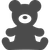Announcements

# Diodes & Thyristors (Si/SiC) Forum DiscussionsLevel 4Level 4

# Power Loss

Hi all,

Could you tell me about the power loss of both TT60N16SOF and TT175N16SOF?

I think that "On-state power loss per arm PTAV = f (ITAV)" described in the data sheet is the condition of frequency 50 / 60Hz.

Do you have this data at 400Hz?

Their application is a three-phase thyristor rectifier.

Best regards,
MS

1 SolutionModeratorModerator

# Re: Power Loss

Hi ,

Thanks for posting in Infineon Community page.

The On state losses for

• TT175N16SOF

VT0 = 0.83 V

rT = 1.3 mΩ

ITAV = 20 A

ITRMS = 34.6 A

PTAV = VT0 • ITAV + rT • I²TRMS

PTAV = (0.83 * 20) + (1.3 * 10^-3 * 34.6 *34.6)

PTAV = 18.156 W

• TT60N16SOF

VT0 = 1 V

rT = 4.8 mΩ

ITAV = 6.7 A

ITRMS = 11.5 A

PTAV = VT0 • ITAV + rT • I²TRMS

PTAV = (1 * 6.7) + (4.8 * 10^-3 * 11.5 *11.5)

PTAV = 7.335 W

To calculate the Reverse recovery losses, use the below formula

E𝑟𝑒𝑐 = 0.5 × 𝑉𝑅𝑝𝑒𝑎𝑘 × 𝑄rr

𝑉𝑅𝑝𝑒𝑎𝑘 =  Peak Reverse Recovery Voltage

𝑄rr = Reverse recovery charge

𝑄rr  depends on the di/dt, which can be selected  from the 𝑄rr vs di/dt graph (Recovered charge Qr = f(-di/dt))

Prec = E𝑟𝑒𝑐 * Frequency

Refer the below images 𝑄rr vs di/dt graphs from the data sheets

Total Power Loss = PTAV  + Prec

Best Regards,

Akhil Kumar

3 RepliesModeratorModerator

# Re: Power Loss

Hello,
Thank you for posting on Infineon Community.

On-State Power loss PTAV is a function of ITAV and conduction angle and does not vary with frequency as shown in datasheet. One can use the given data for any frequency for average on-state losses.
The average value of the on-state loss PTAV Is calculated according to the following formula:
PTAV = VT(TO) • ITAV + rT • I²TRMS
where,
ITAV :  Mean Value of On-State Current
rT : On-State Resistance
VT(TO) : Threshold Voltage
ITRMS: R.M.S value of On-State Current

For total power loss calculations, please let me know about the current and voltage ratings required in your application.

Thanks and Regards,
AnshikaLevel 4Level 4

# Re: Power Loss

Hi,

The current and voltage are as follows.

1.TT175N16SOF

ITAV = 20A

ITRMS = 34.6A

V = 250Vac (RMS value of AC input voltage)

2.TT60N16SOF

ITAV = 6.7A

ITRMS = 11.5A

V = 250Vac (RMS value of AC input voltage)

Best regards,
MSModeratorModerator

# Re: Power Loss

Hi ,

Thanks for posting in Infineon Community page.

The On state losses for

• TT175N16SOF

VT0 = 0.83 V

rT = 1.3 mΩ

ITAV = 20 A

ITRMS = 34.6 A

PTAV = VT0 • ITAV + rT • I²TRMS

PTAV = (0.83 * 20) + (1.3 * 10^-3 * 34.6 *34.6)

PTAV = 18.156 W

• TT60N16SOF

VT0 = 1 V

rT = 4.8 mΩ

ITAV = 6.7 A

ITRMS = 11.5 A

PTAV = VT0 • ITAV + rT • I²TRMS

PTAV = (1 * 6.7) + (4.8 * 10^-3 * 11.5 *11.5)

PTAV = 7.335 W

To calculate the Reverse recovery losses, use the below formula

E𝑟𝑒𝑐 = 0.5 × 𝑉𝑅𝑝𝑒𝑎𝑘 × 𝑄rr

𝑉𝑅𝑝𝑒𝑎𝑘 =  Peak Reverse Recovery Voltage

𝑄rr = Reverse recovery charge

𝑄rr  depends on the di/dt, which can be selected  from the 𝑄rr vs di/dt graph (Recovered charge Qr = f(-di/dt))

Prec = E𝑟𝑒𝑐 * Frequency

Refer the below images 𝑄rr vs di/dt graphs from the data sheets

Total Power Loss = PTAV  + Prec

Best Regards,

Akhil Kumar